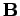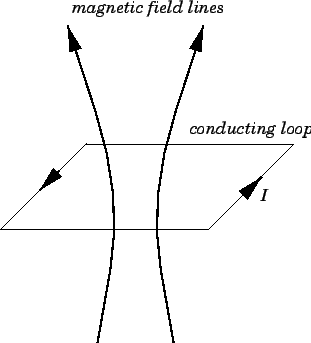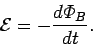Next: Magnetic Induction Up: Magnetic Induction Previous: Faraday's Law

## Lenz's Law

We still have not specified in which direction the emf generated by a time-varying magnetic flux linking an electric circuit acts. In order to help specify this direction, we need to make use of a right-hand rule. Suppose that a currentcirculates around a planar loop of conducting wire, and, thereby, generates a magnetic field. What is the direction of this magnetic field as it passes through the middle of the loop? Well, if the fingers of a right-hand circulate in the same direction as the current, then the thumb indicates the direction of the magnetic field as it passes through the centre of the loop. This is illustrated in Fig. 34.Consider a plane loop of conducting wire which is linked by magnetic flux. By convention, the direction in which current would have to flow around the loop in order to increase the magnetic flux linking the loop is termed the positive direction. Likewise, the direction in which current would have to flow around the loop in order to decrease the magnetic flux linking the loop is termed the negative direction. Suppose that the magnetic flux linking the loop is increased. In accordance with Faraday's law, an emf is generated around the loop. Does this emf act in the positive direction, so as to drive a current around the loop which further increases the magnetic flux, or does it act in the negative direction, so as to drive a current around the loop which decreases the magnetic flux? It is easily demonstrated experimentally that the emf acts in the negative direction. Thus:

The emf induced in an electric circuit always acts in such a direction that the current it drives around the circuit opposes the change in magnetic flux which produces the emf.
This result is known as Lenz's law, after the nineteenth century Russian scientist Heinrich Lenz, who first formulated it. Faraday's law, combined with Lenz's law, is usually written(196)

The minus sign is to remind us that the emf always acts to oppose the change in magnetic flux which generates the emf.Next: Magnetic Induction Up: Magnetic Induction Previous: Faraday's Law
Richard Fitzpatrick 2007-07-14